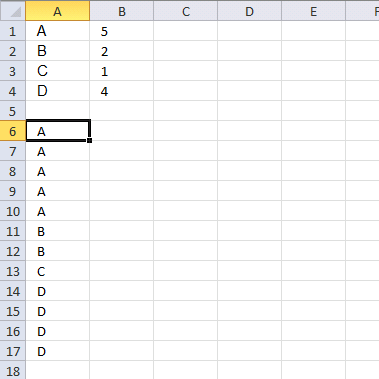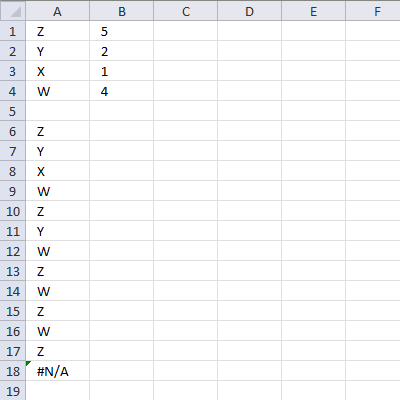Author: Oscar Cronquist Article last updated on August 30, 2017

I failed to find right article in your blog and therefore I want to ask you in newest post. So I have table similar like this:
A 5
B 2
C 1
D 4
Is it possible with formula to generate list like this:
A
A
A
A
A
B
B
C
D
D
D
D
Thank you in advance!Array formula in cell A6:

=INDEX(\$A\$1:\$A\$4,MATCH(FALSE, COUNTIF(\$A\$5:A5,\$A\$1:\$A\$4)=\$B\$1:\$B\$4,0))

### Explaining array formula in cell A6

Step 1 - Count previous values

COUNTIF(\$A\$5:A5,\$A\$1:\$A\$4)

becomes

COUNTIF("",{"A";"B";"C";"D"})

and returns {0;0;0;0}

Step 2 - Compare array values to list

COUNTIF(\$A\$5:A5,\$A\$1:\$A\$4)=\$B\$1:\$B\$4

becomes

{0;0;0;0}={5;2;1;4}

and returns {FALSE;FALSE;FALSE;FALSE}

Step 3 - Match first FALSE value

MATCH(FALSE, COUNTIF(\$A\$5:A5,\$A\$1:\$A\$4)=\$B\$1:\$B\$4,0)

becomes

MATCH(FALSE, {FALSE;FALSE;FALSE;FALSE},0)

and returns 1.

Step 4 - Return a value of the cell at the intersection of a particular row and column

=INDEX(\$A\$1:\$A\$4,MATCH(FALSE, COUNTIF(\$A\$5:A5,\$A\$1:\$A\$4)=\$B\$1:\$B\$4,0))

becomes

=INDEX(\$A\$1:\$A\$4,1)

and returns A in cell A6.

I have made an Advanced Excel Course if you want to learn how to construct powerful array formulas.

### Repeat the range according to criteria in loop

Hi Oscar,
Great Job.. Is this possible to repeat the range according to criteria in loop.. like below.Z 5
Y 2
X 1
W 4then Z 5 Times, Y 2 Times.... but in series
Z
Y
X
W
Z
Y
W
Z
W
Z
W
ZRegards,
DebArray formula in cell A6:

=INDEX(\$A\$1:\$A\$4, MATCH(MIN(IF(COUNTIF(\$A\$5:A5, \$A\$1:\$A\$4)=\$B\$1:\$B\$4, "", COUNTIF(\$A\$5:A5, \$A\$1:\$A\$4))), IF(COUNTIF(\$A\$5:A5, \$A\$1:\$A\$4)=\$B\$1:\$B\$4, "A", COUNTIF(\$A\$5:A5, \$A\$1:\$A\$4)), 0))